### IMO Shortlist 1984 problem 4

Kvaliteta:
Avg: 0,0
Težina:
Avg: 0,0
Let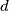$d$ be the sum of the lengths of all the diagonals of a plane convex polygon with$n$ vertices (where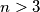$n>3$). Let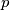$p$ be its perimeter. Prove that: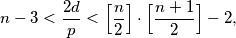where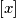$[x]$ denotes the greatest integer not exceeding$x$.
Izvor: Međunarodna matematička olimpijada, shortlist 1984